# Sample JEE(MAIN) Mathematics Mock Test

## 30 Questions MCQ Test JEE Main Mock Test Series 2021 & Previous Year Papers | Sample JEE(MAIN) Mathematics Mock Test

Description
This mock test of Sample JEE(MAIN) Mathematics Mock Test for JEE helps you for every JEE entrance exam. This contains 30 Multiple Choice Questions for JEE Sample JEE(MAIN) Mathematics Mock Test (mcq) to study with solutions a complete question bank. The solved questions answers in this Sample JEE(MAIN) Mathematics Mock Test quiz give you a good mix of easy questions and tough questions. JEE students definitely take this Sample JEE(MAIN) Mathematics Mock Test exercise for a better result in the exam. You can find other Sample JEE(MAIN) Mathematics Mock Test extra questions, long questions & short questions for JEE on EduRev as well by searching above.
QUESTION: 1

### y-axis divides the segment joining points (-3,-4) and (1,-2) in the ratio

Solution: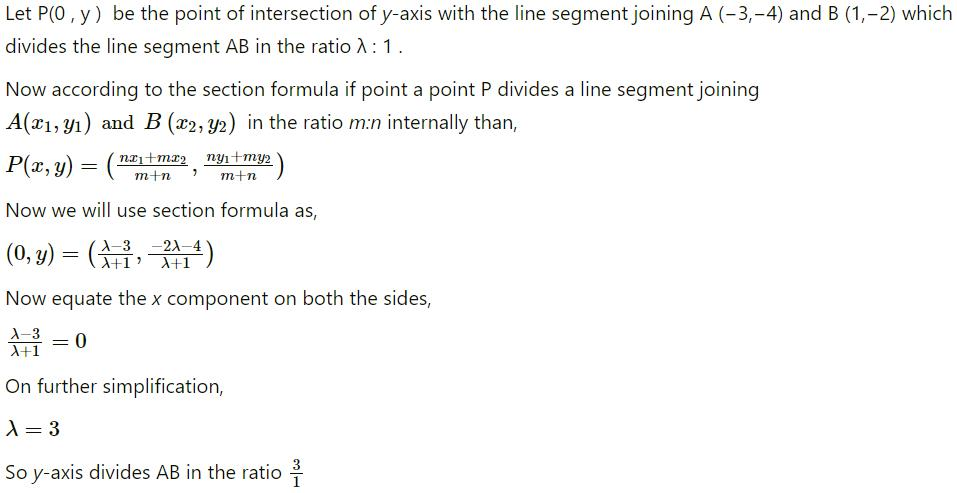QUESTION: 2

### A pair of tangent lines are drawn from the origin to the circle x2+y2+20(x+y)+20 = 0. The equation of pair of tangents is

Solution: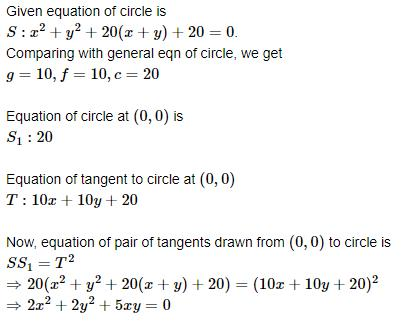QUESTION: 3

### If the polar w.r.t.y2 = 4ax touches the ellipse (x2/a2) + (y2/b2) = 1, the locus of its pole is

Solution:

Let coordinates of the pole be (h,k) then equation of the polar of y2=4ax is ky=2a(x+h)

y=(2a/k)x+(2ah)/K

Since line (1) is touching the ellipse

x2/a2+y2/b2=1

4a2b2/k2=a2.4a2/K2+b2

Required locus is 4a2x2=4a4+b2y2

=> 4a2x2b2y2=4a4

Hence A is the correct answer.

QUESTION: 4

If ω = (-1 + √3i)/2,then (3 + ω + 3ω2)4 is

Solution:

(3+w+3w²)4 = [(1+w+w²)+2w²+2]4= 24(w²+1)=16(-w)4 = 16w4= 16w

QUESTION: 5

The area of the curve |x|+|y|=4 is

Solution:

If you draw a graph it will be clear let us consider 4 coordinates (4,0),(-4,0),(0,-4),(0,4) if you join this points you will get a square.this square can again be divided into 4 symmetric triangles.therefore the area = 4 times area of one triangle

area of triangle = 1/2bh=1/2*4*4=8

therefore total area = 4*8=32

QUESTION: 6

If f(x) = sinx-(x/2) is increasing function, then

Solution:
QUESTION: 7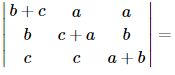Solution:
QUESTION: 8

The principal value of sin⁻1(sin 2π/3) is

Solution:

Sine function is a periodic function. Its value repeat after an interval of 2π. Since 2π/3 is out of its inverse principle range (which is from -π/2 to π/2) so we will write sin 2π/3 = sin π/3. Now its in the principle range. Therefore sin⁻1(sin 2π/3) = sin⁻1(sin π/3) = π/3

QUESTION: 9

The equation of the curve passing through the origin and satisfying the differential equation dy/dx = (x − y)2 is

Solution:

We have, dy/dx=(x−y)2
Let (x−y)=v. Then,
1−dy/dx=dv/dx⇒dy/dx=1−dv/dx
∴ dy/dx=(x−y)2
⇒ 1−dv/dx=v2
⇒ 1−v2=dv/dx
⇒ dx=1/1−v2dv
⇒ 2∫dx=2∫1/1−v2dv
⇒ 2x=log(1+v/1−v)+logC
⇒ C(1+v/1−v)=e2x
⇒C(x−y+1/y−x+1)=e2x⇒C(x−y+1)=e2x(y−x+1)
Taking C = 1, we find that option (a) is correct.

QUESTION: 10

In the following question, a
Statement-1 is given followed by a corresponding
Statement-2 just below it. Read the statements carefully and mark the correct answer-

Consider the system of equations
ax+by = 0, cx+dy = 0, where a, b, c, d ∈ {0,1}
Statement-1: The probability that the system of equations has a unique solution is 3/8 .
Statement-2: The probability that the system of equations has a solution is 1.

Solution:

For unique solution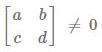where a, b, c, d {0, 1}

total cases = 16
Favorable cases = 6 (either ad =1, bc = 0 or ad = 0, bc =1).

Probability that system of equation has unique solution is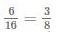and system of equations has either unique solution or infinite solutions so that probability for system to have a solution is 1.

QUESTION: 11

The maximum area of the rectangle that can be inscribed in a circle of radius r is

Solution:
QUESTION: 12

If z ≠ 0 is a complex number such that Arg (z) = π/4, then

Solution:
QUESTION: 13

In the following question, a Statement of Assertion (A) is given followed by a corresponding Reason (R) just below it. Read the Statements carefully and mark the correct answer-
Assertion (A): Angle between the vectors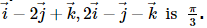Reason (R): If θ is the angle between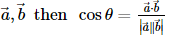Solution:

Solution :- A = i^+ j^ and B = j^+k^

A = (2)^1/2 , B = (2)^1/2

​θ=(cos^−1)(A.B/AB)=(cos^−1)(1/2).

or, θ=(cos^−1)(cosπ/3) = π/3

Therefore, both Assertion and Reason are correct and Reason is the correct explanation for Assertion.

QUESTION: 14

The number of distinct permutations of the letters of the word STATISTICS that begin and end with the letter S is

Solution:
QUESTION: 15

If A and B are two events and B is a subset of A, then P(A/B) is equal to

Solution:

Since, B is a subset of A so B ∩ A = B
now, P(A|B) = P(A ∩ B)/P(B) = P(B)/P(B) = 1

QUESTION: 16

If A and B are such events that P(A)>0 and P(B)≠1, then P(A̅/B̅) is equal to

Solution:
QUESTION: 17

If x2 + px + q is the quadratic equation whose roots are a - 2 and b - 2 where a and b are the roots of x2 - 3x + 1 = 0, then

Solution:
QUESTION: 18

The A.M., H.M. and G.M. between two numbers are 144/15, 15 and 12, but not necessarily in this order. Then H.M., G.M. and A.M. respectively are

Solution:

A.M. ,G.M., H.M. between two numbers are 144/15 , 15 , 12.

We know that A.M > G.M > H.M

So, the order is

Therefore, A.M  = 15 ,    G.M = 12 ,    H.M = 144/15

Then,

H.M, G.M and A.M = 144/15 ,12, 15

QUESTION: 19

The measure of dispersion is

Solution:

Standard deviation (SD) is the most commonly used measure of dispersion. It is a measure of spread of data about the mean. SD is the square root of sum of squared deviation from the mean divided by the number of observations.

QUESTION: 20

If lines y = 3x+1 and 2y = x+3, are equally inclined with y = mx+4, m =

Solution:

Given,
y = 3x + 1 , slope of it {m1} = 3
2y = x + 3 , slope of it {m2} = 1/2
angle between y = 3x + 1 and y = mx + 4 is ∅
then , tan∅ = |3 - m|/| 1 + 3m| ____(1)

it is given that angle between the lines 2y = x + 3 and y = mx + 4 is also ∅ .
then, tan∅ = |1/2 - m|/| 1 +m/2|________(2)
from equations (1) and (2),
|3 - m|/|1 + 3m| = | 1/2 - m|/| 1 + m/2|
|3 - m|/|1 + 3m| = |1 - 2m|/|2 + m|
taking positive sign ,
(3 - m)(2 + m) = (1 - 2m)( 1 + 3m)
6 + 3m -2m -m^2 = 1 + 3m -2m - 6m^2
5m^2 = -5
m^2 = -1 it's not possible .
taking negative sign,
(3 - m)(2 + m) = -(1 - 2m)(1 + 3m)
6 + 3m - 2m - m^2 = -1 - 3m + 2m + 6m^2
7m^2 - 2m -7 = 0
m = { 2 ± √(4 + 49 × 4)}/14
= { 2 ± 2√50}/14
= { 1 ± √50}/7
= {1 ± 5√2}/7
hence, m = { 1 ± 5√2}/7

QUESTION: 21

The direction ratios of the diagonals of a cube which joins the origin to the opposite corner are (when the 3 concurrent edges of the cube are co-ordinate axes)

Solution:

Let the length of the sides of cube is a, then coordinates of corner (P) opposite to origin are (a,a,a)

∴  Direction ratios of diagonal OP are (a−0,a−0,a−0)

∴(a,a,a)i.e.(1,1,1)

QUESTION: 22

The points (1, 2, 3), (-1, -1, -1) and (3, 5, 7) are the vertices of

Solution:

Explanation:- Using distance formula

AB=√29. BC=√116=2√29

AC=√29

BA+AC=BC

The points are collinear, so it doesn't make any triangle.

QUESTION: 23

cosA+sin(270°+A)-sin(270°-A)+cos(180°+A) =

Solution:

Sin (180) = 0  cos (180) = - 1  sin (270) = - 1  cos (270) = 0  ∴ LHS  = cosA + sin (270 + A) - sin (270 - A) + cos (180 + A)  = cosA + sin(270)cos(A) + cos(270)sin (A) - [sin (270)cos (A) - cos (270)sin (A)] + cos (180)cos (A) - sin (180)sin (A)  = cos(A) + 2cos (270)sin (A) - cosA  = 0  = RHS

QUESTION: 24

The number of vectors of unit length perpendicular to vectors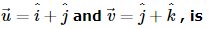Solution:

Since the first vector is in x-y plane and other is in y-z plane so if the resultant 2 vector seen as a plane then then perpendicular to them can be in both front and back

QUESTION: 25

Define a real valued function of a real variable given by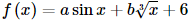where a and b are real numbers. f(log10(log5 10)) = 7, then the value of f(log10(log10 5)), is

Solution:
QUESTION: 26

The value of λ for which the area bounded between y = x2 - 1 and y = λ x + |x| is bisected by the y-axis is

Solution:
QUESTION: 27

Let B and C lie on the circle with OA as a diameter, where O is the origin. If ∠ AOB = ∠ BOC = θ and z1, z2, z3 representing the points A, B, C respectively, then which one of the following is true?

Solution:
QUESTION: 28

In the following question, a Statement of Assertion (A) is given followed by a corresponding Reason (R) just below it. Read the Statements carefully and mark the correct answer-

Assertion(A):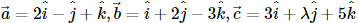and if these vectors be coplanar, then λ is -8.
Reason(R):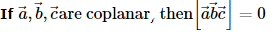Solution:
QUESTION: 29

In the following question, a Statement of Assertion (A) is given followed by a corresponding Reason (R) just below it. Read the Statements carefully and mark the correct answer-
Assertion(A): The orthocentre of a given triangle is coincident with the incentre of the pedal triangle of the given triangle.
Reason(R): Pedal triangle is the ex-central triangle of the given triangle.

Solution:
QUESTION: 30

In the following question, a Statement of Assertion (A) is given followed by a corresponding Reason (R) just below it. Read the Statements carefully and mark the correct answer-
Assertion(A): Area common to the curve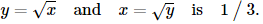Reason(R):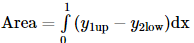Solution: